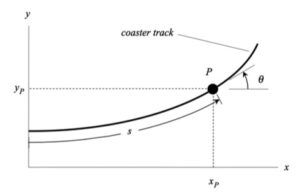# Homework H1.D - Sp23

 Problem statement Solution videoDISCUSSION:

## 28 thoughts on “Homework H1.D - Sp23”

1.Idris Ismail Muhammad says:

Is anyone else getting the wrong link for HW 1.D?

1.Katie-Jane Hollar Klembarsky says:

Yes, my H1.D problem statement shows the same problem as H1.C.

1.Idris Ismail Muhammad says:

If you go to daily schedule it gives the right one

2.William Grant Dierking says:

Do we write our answers in terms of i and j or eT and eN? If the latter is true, do we just not use the given theta in our calculations?

1.Bernardo de Barros Barreto C Machado says:

I think we’re supposed to leave the answer in terms of eT and eN. Second part I am not sure

2.Lucas James Ford says:

I did it by finding the unit vectors eT and eN in terms of theta and then simplifying theta in terms of s, and that way you can get the entire equation for v and a in terms of s.

1.Sam Plumer says:

How do you write et and en in terms of theta? I can't find any equations relating them directly.

2.Arul Verma says:

Ok so after plugging in theta, I can get a simplified equation for the magnitude of acceleration. But, how do you go about finding ds/dt, as that is the only unknown left in
a = v_dot * e_t + (v^2/ρ) * e_n ?

3.William Jackson Baun says:

We leave it in terms of eT and eN as when it says "path variable components" I believe that is what that refers to.

4.Sharon Ni says:

On page 34 of the lecturebook, it has a formula that you can use to change the eT and eN to i and j components, and one of the TAs I asked in the help room also said that they thought the problem is asking for this way instead of leaving it in eT and eN.

5.Luis Martinez says:

My thinking is that we do leave part a in terms of et and en but in part b after we plug in the given value of s then we would transform it to cartesian coordinates using the angle given to make the sketch on the xy plane.

3.Ryan Andrew Robinson says:

So, is it confirmed that our answers should be in Cartesian coordinates? The answer I got for acceleration is very long so I'm not sure if I am doing it right. I also didn't need curvature.

4.adaetz says:

When differentiating to find v_dot, I took dv/ds from the speed equation and then multiplied it by v = v(s). Is this correct? Is v in the equations for finding v_dot and acceleration the same v as v(s)?

1.Audrey says:

v_dot should equal dv/dt, since acceleration (v_dot) is the time derivative of velocity. By using the chain rule, dv/dt = (dv/ds)*(ds/dt) = v*(dv/ds).

2.Audrey says:

Yes, v in the equations for finding v_dot and acceleration is the same v as v(s).

3.Kailin Yang says:

Yes, that is correct cause dv/dt=dv/ds * ds/dt (V(s)).

5.Allie says:

Are we able to just find v, v_dot, and ρ and plug them into the acceleration equation a = v_dot * e_t + (v^2/ρ) * e_n ? This is the only way I can figure out how to find the acceleration vector, but then the equation for theta is completely unused. I don't understand, though, how to find the acceleration in terms of s with theta and not in terms of t like the steps on page 34 show.

1.Audrey says:

I also solved it this way. I don't believe that the equation for theta is used, unless we are required to change the final answers from e_t and e_n into i and j coordinates. There doesn't seem to be a final consensus on what coordinate system to leave the answer in.

6.Madeline B says:

How can you determine the curvature? We don't have a radius nor exact terms of x_p and y_p, I am not sure where to begin with this.

1.Allie says:

The curvature is given as p = 1/bs in the problem statement. It varies with the distance since it doesn't have a constant radius like a circle.

1.Madeline B says:

But how do we use this equation? Do we need to use it with arc length to calculate s?

1.Zachary William Delahunty says:

The "p = 1/bs" is what you plug into the acceleration equation "a = v(dot)et + (v^2/p)en"

7.Liam Quentin Johnson says:

For part a.) should we leave the symbols for the constants in our equations or substitute in the actual numbers?

1.John D Dec says:

I believe for the final answer you should substitute the numbers into your equations, however when working out the problem you should keep the problem symbolic.

8.Elijah Allan Collins says:

Did anyone keep their answer in En and Et notation? I compared my answer with someone who converted theirs to i and j notation and the En and Et notation were much more simple. However, there was no need for theta anymore. Any insights on this would be appreciated. Thanks.

1.Allie says:

I think we're supposed to keep it in en and et notation because they want the answer in path coordinates, not cartesian ones.

9.Allie says:

Are we required to use the equation for theta? I got an answer, but never used that equation. I could not figure out how to work that equation into my solution. Is there a way to solve this without using it?

1.Alex L says:

I think you only need the equation for theta whenever you're going from path to cartesian and because we don't have to do that for this question, you just don't use the equation for theta